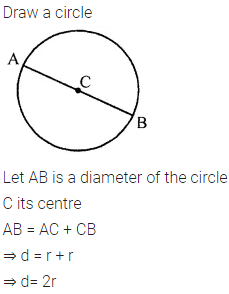# ML Aggarwal Class 6 Solutions for ICSE Maths Chapter 9 Algebra Ex 9.2

## ML Aggarwal Class 6 Solutions for ICSE Maths Chapter 9 Algebra Ex 9.2

Question 1.
If the side of an equilateral triangle is l, then express the perimeter of the triangle in terms of l.
Solution: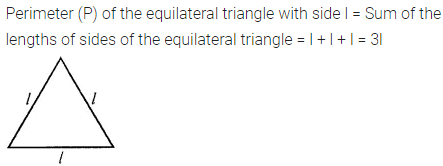Question 2.
The side of a regular hexagon is l. Express its perimeter in terms of l.
Solution: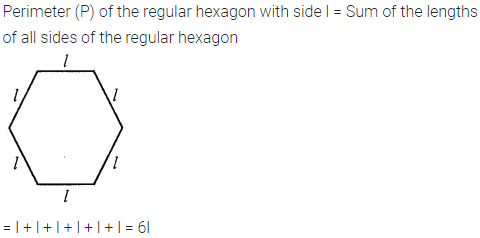Question 3.
The length of an edge of a cube is l. Find the formula for the sum of lengths of all the edges of the cube.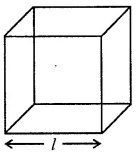Solution: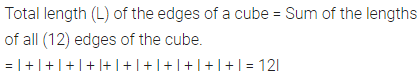Question 4.
If the radius of a circle is r units, then express the length of a diameter of the circle in terms of r.
Solution: